In this example, we will investigate the issues at high data bit rate, particularly 10 Gbps (OC-192) systems in metro area ring networks. At higher bit rates, in addition to loss compensation, we may also need to consider the chromatic dispersion compensation. Different options related to dispersion and initial chirp will be investigated.

For this case, the receiver sensitivity is -16 dBm which reduces the allowable loss to Lallowable = 3-(-16) = 13dB. Assuming a power of 3 dBm per channel, the amount of amplification needed in the ring is Grequired = -(3×13.5-3×3.8-(-16)-3) = 35.9 dB. We can again use the unity gain segment approach for power compensation. By using this approach, migration from 2.5 Gbps to 10 Gbps is easily done in terms of power budgeting since the total loss is not changed, even though the allowable loss is changed. In this case, the received power will be well above the receiver sensitivity. See “Unity Gain Design” on page 390.

In the case of directly modulated DFB lasers, the limiting transmission distance is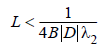where λ2 is root-mean-square spectral width, with a typical value of 0.15 nm for D=16ps/(km-nm) and 2.5 Gbps, and L ≈ 42km.

For externally modulated sources, the limiting transmission distance is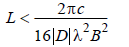with D=16ps/(km-nm) and 2.5 Gbps, L ≈ 500km. Therefore, Group Velocity Dispersion (GVD) is not a limiting effect for a 2.5 Gbps communications network.

## No dispersion compensation

At high bit rates, GVD may become a limiting factor for the transmission distance. When the bit rate increased to 10 Gbps, GVD-limited transmission distance drops to 30 km for externally modulated sources and to 10.5 km for directly modulated sources. Figure 1 shows the eye diagram when the bit rate is 10 Gbps and transmitter power is 3 dBm/channel and directly modulated sources are used. Ever increasing transmitter power does not open the eye. In fact, the shape of the eye shows that pulse direction distortion is related to dispersion. In this example, we have used the unity gain segment approach, which means that loss in each fiber span and node is compensated immediately at the following node. This project is given in Migrating to 10 Gbps no dispersion compensation.osd file.

For simplicity, in this project we have used the “Directly Modulated Laser Measured” model for directly modulated sources and set the alpha parameter to -5.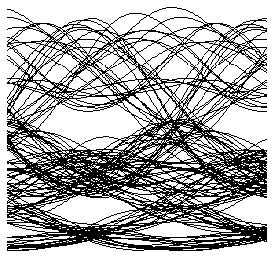Figure 1: Eye diagram of channel 1 at node 4 when the transmitter power is 3 dBm and bit rate is 10 Gbps

Therefore, at 10 Gbps bit rate, you have to use dispersion compensation. Total dispersion per 50 km span is around 16×50 = 800 ps / nm. This will require 10.25 km of dispersion compensating fiber (DCF) with D = -80 ps / nm / km for each fiber span. There are several avenues to compensate for the accumulated dispersion that we will investigate in the following sections.

## “Per span” dispersion compensation

DCF can be used after each span, or total accumulated dispersion can be compensated at a certain point. To preserve the scalability of the network, and keeping in mind the dynamic structure of the metro traffic, it seems to be the best choice to distribute the dispersion compensation to every node. This will also ensure that dispersion experienced by dynamically routed signals will be compensated properly. The project is found in the Migrating to 10 Gbps dispersion compensation at each node.osd file. Figure 2 shows the network layout when unity gain approach and per node dispersion compensation is used.

In this project, we again used directly modulated transmitters as described in previous sections. To find the optimum length for dispersion compensation, we swept the length of the DCFs from 13 km to 18 km. Figure 4 shows the simulation results in terms of Q factor when transmitter power is 3 dBm. As you can see from this figure, maximum Q factor is observed when the length of DCFs is about 18 km. You can also identify from this figure that there is ±2 km of tolerance for each DCF for a minimum Q factor of 6. Figure 5 shows the eye diagram when maximum Q factor is observed.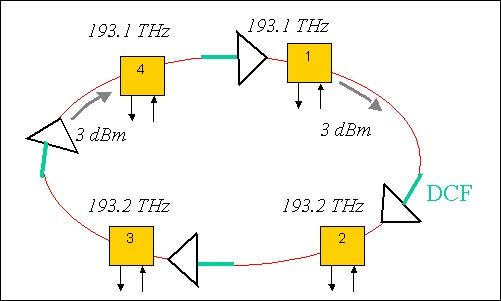Figure 2: Ring network layout when unity gain approach and per span dispersion compensation is used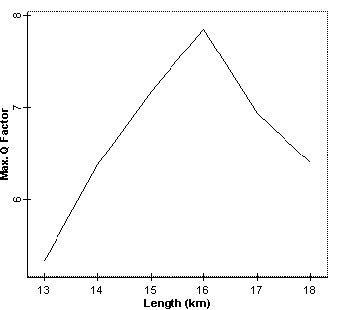Figure 3: Q factor vs. DCF length when directly modulated transmitter is used

To investigate the effect of transmitter type, we have replaced directly modulated sources with externally modulated ones. The project is given in the Migrating to 10 Gbps dispersion compensation at each nodeEM.osd file. The results are shown in Figure 5 in terms of Q factor for different DCF lengths. Maximum Q factor is observed when the length of DCF is about 14 km. Compared to the directly modulated transmitter case, this curve is broader and dispersion compensation tolerance is higher; 14 km for this case, which is related to initial chirp on the signal. The influence of the initial chirp on system performance will be investigated in the Negative dispersion fiber for Metro networks.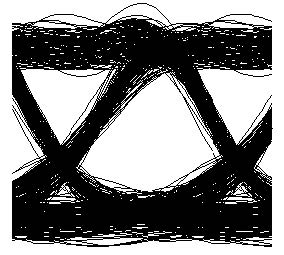Figure 4: Eye diagram at node 4 when per span dispersion compensation is used and DCF length is 16 km

## “Lumped” dispersion compensation

In the “lumped” dispersion compensation case, one DCF and an extra amplifier is inserted just before node 2 to compensate the total dispersion. This project is found in the Migrating to 10 Gbps lumped dispersion compensation.osd file. Figure 5 shows Q factor versus DCF length at node 4 when directly modulated laser is used and transmitter power is 3 dBm. As can be seen from this figure, this configuration definitely improves the network performance compared to the uncompensated case, but is worse than the case with distributed compensation.

In the case of lumped dispersion compensation, DCF length tolerance is about ±1.5 where maximum Q factor is observed, DCF is 45 km, and maximum Q factor is about 6.2. Furthermore, this type of dispersion compensation is not suitable for metro networks in which the wavelength routing path cannot be estimated. For example, consider the path recovery schemes in a much more complex network with more than one ring. Depending on the structure of the network, one particular wavelength can follow a path that does not pass over the single dispersion compensation fiber in the network.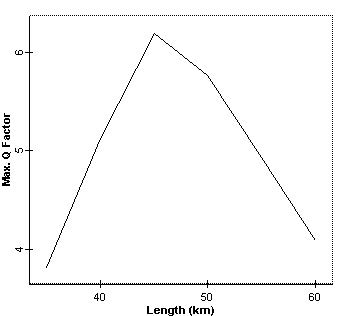Figure 5: Q factor versus DCF length at node 4 when “lumped” dispersion compensation is used

References:
 G. P. Agrawal, Fiber-Optic Communication Systems, Wiley-Interscience, 1997.

 R. Ramaswami and K. N. Sivarajan, Optical Networks: A practical Perspective, Morgan Kaufmann, 1998.